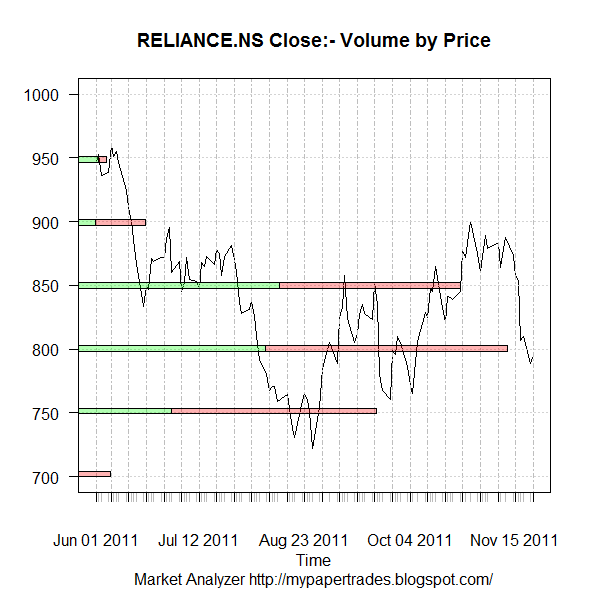Want to share your content on R-bloggers? click here if you have a blog, or here if you don't.

R-Bloggers is a wonderful site which offers some great ideas for analysis.

While I have been busy of late, hence could not do much with R, I was inspired by this post by Eric Nguyen on Volume by Price chart.

This chart can be used with a great effect in ones trading strategies. It helps in identifying the support and resistance zones, and a breakout from these levels used as entry/exit points

Extending the work done by Eric, and making few changes in his original code, here is what I have achieved.Reliance EOD Volume by Price chart

It can now be visually observed the role of volume in providing support/resistance zones.

Future Work: Make animation-like effect to show case the strength offered by volumes at various price levels over a period of time.

Not the best way to code, here is the workable version for more experimentation and extension

```library(quantmod)
###############################################################

#Original Code
#http://blog.datapunks.com/2011/10/volume-by-price-charts-with-r-first-attempt/

#Change the ticker to get chart of any "yahoo" symbol
ticker = "RELIANCE.NS"
symbol <- getSymbols(ticker)
stock <- xts(get(symbol))

#remove stock name
names(stock)[names(stock)==paste(symbol,'.Open',sep="")] <- 'Open'
names(stock)[names(stock)==paste(symbol,'.Close',sep="")] <- 'Close'
names(stock)[names(stock)==paste(symbol,'.Volume',sep="")] <- 'Volume'
names(stock)[names(stock)==paste(symbol,'.High',sep="")] <- 'High'
names(stock)[names(stock)==paste(symbol,'.Low',sep="")] <- 'Low'

stock\$posVbP <- Vo(stock[which(Lag(Cl(stock)) <= Cl(stock))])
stock\$negVbP <- Vo(stock[which(Lag(Cl(stock)) > Cl(stock))])

#Since NAs got generated, replace NAs with 0.
stock[is.na(stock)] <- 0

#Subset for data since May 2011
myQ <- stock['2011-06::']

#Define function to add positive and negative volumes by prices
pVolBlock <- function(x) sum(myQ\$posVbP[myQ\$t==x])
nVolBlock <- function(x) sum(myQ\$negVbP[myQ\$t==x])

funcPriceByVol <- function(x){
myDiv <- 50         #Divisor for stock
myQHi <- as.integer(ceiling(max(Cl(myQ))/myDiv)*myDiv)  #Identify High of Series
myQLo <- as.integer(floor(min(Cl(myQ))/myDiv)*myDiv) #Identify Low of Series

myBreaks <- as.integer(seq(myQLo, myQHi, by=myDiv))  #Create Breaks of interval divisor

# Identify and assign price intervals
myQ\$t <<- myBreaks[findInterval(myQ\$Close,myBreaks,all.inside=T)]

myVolsP <- unlist(lapply(myBreaks,pVolBlock)) #Add Positive Volumes to block
myVolsN <- unlist(lapply(myBreaks,nVolBlock)) #Add Negative Volumes to block
myVols <- rbind(myVolsP,myVolsN)   #Bind the Positive and Negative Volumes
colnames(myVols) <- myBreaks    #Define Column Naes
x=myVols
}

myPBV <- funcPriceByVol()

#lets Plot the graph now

plot(Cl(myQ),yaxt="n", ylab="",xlab="Time",
ylim=c(min(as.integer(colnames(myPBV))),max(as.integer(colnames(myPBV)))),
main=paste(ticker, "Close:- Volume by Price"),sub="Market Analyzer http://mypapertrades.blogspot.com/")
axis(side=2,las=1)
par(new=T)
barplot(height=myPBV,
beside=F,horiz=T, col=c(rgb(0,1,0,alpha=.3),rgb(1,0,0,alpha=.3)),
xlim=c(0,max(myPBV[1,]+myPBV[2,])*1.1),
space=10, width=3,xaxt="n",yaxt="n",
las=2)```